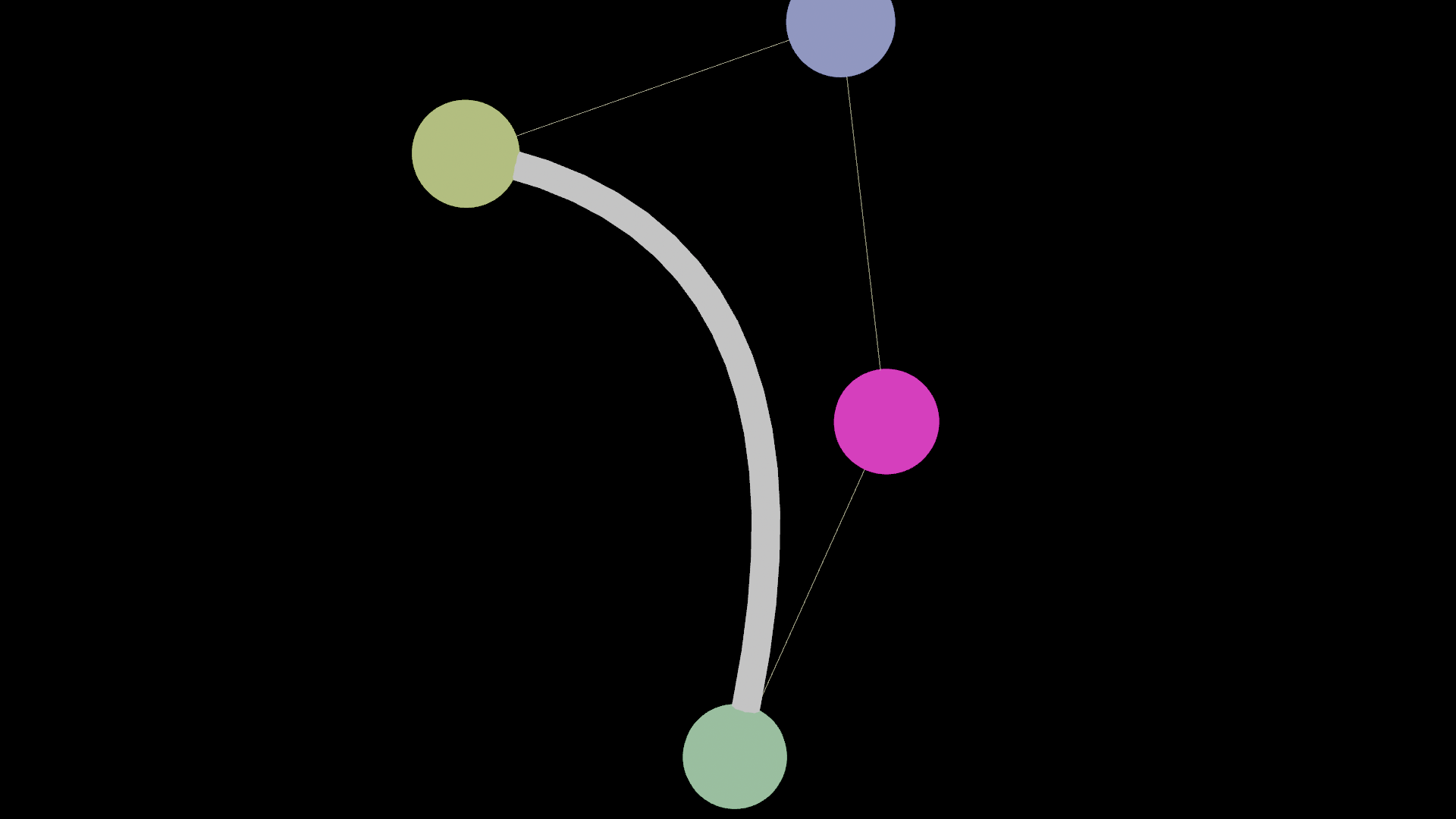# Cubic bézier curves in VEX

calendar_today

24.05.2022

label

VEX, Modeling

mouse

17.0

### 1 Drawing a spline

A cubic bezier curve linearly interpolates between 4 point positions. The first interpolation between point 0 and 1 and the second between 2 and 3. Then it interpolates between the first and the second interpolation.

Thus resulting in a smooth curve that starts at point 0, ends at point 3 and bends towards point 1 and 2. In this code the linear interpolations are driven by `u`. A for loop is adding a new point at the calculated positions.

``````int steps = chi('steps');

vector pos_0 = point(1, "P", 0);
vector pos_1 = point(1, "P", 1);
vector pos_2 = point(1, "P", 2);
vector pos_3 = point(1, "P", 3);

for(int i = 0; i < steps; i++) {

float u = i / float(steps - 1);
vector pos_a = lerp(pos_0, pos_1, u);
vector pos_b = lerp(pos_2, pos_3, u);
vector pos = lerp(pos_a, pos_b, u);